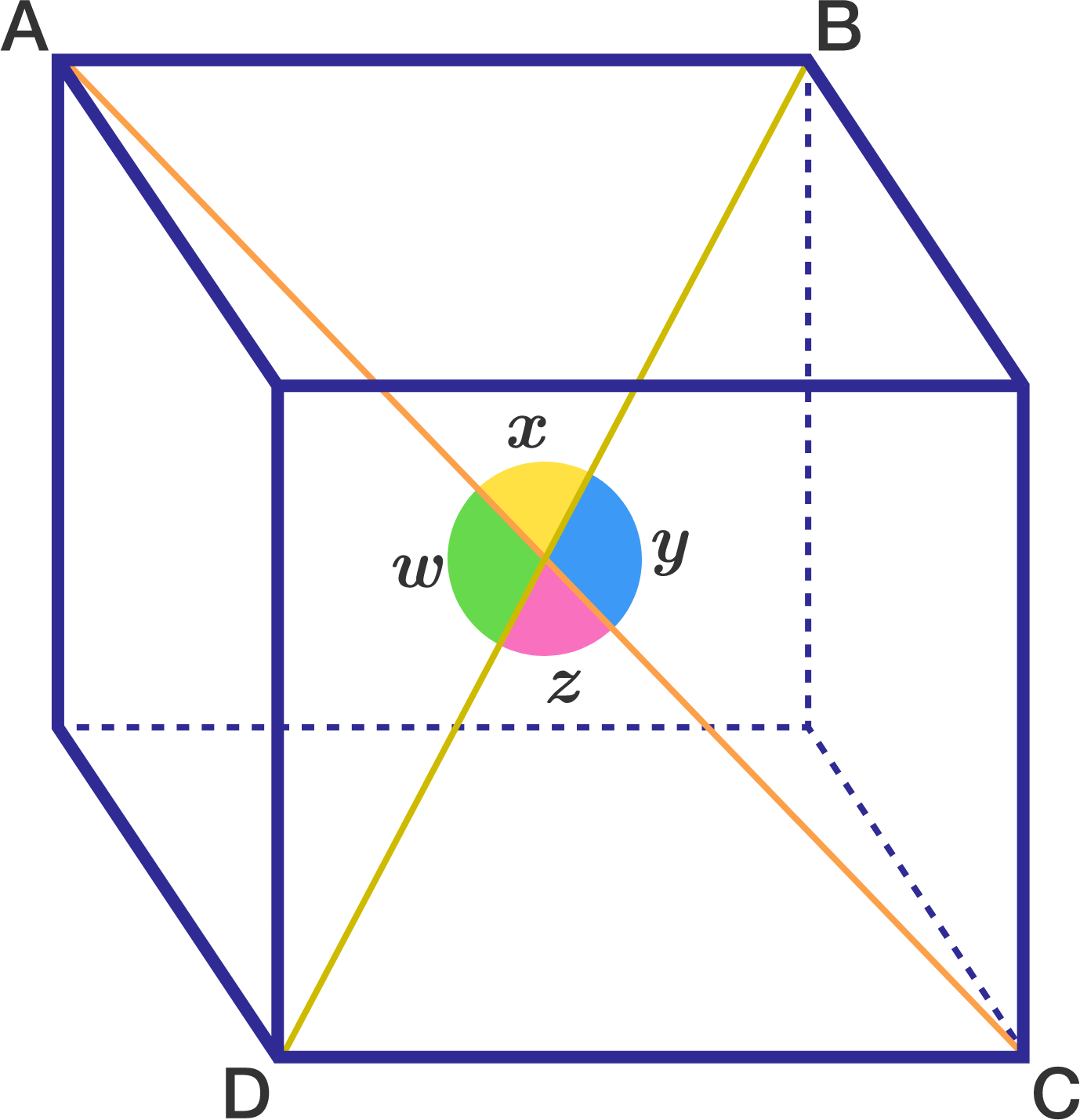# Revival Of This Problem. Part 1For the cube shown above, let

$v = \cos^2 w + \cos^2 x + \cos^2 y + \cos^2 z.$

If $v$ is in the form $\frac{c}{d}$, where $c$ and $d$ are coprime positive integers, find $c+d$.

Note: $w$, $x$, $y$ and $z$ are all angles between 2 diagonals of the cube.

×